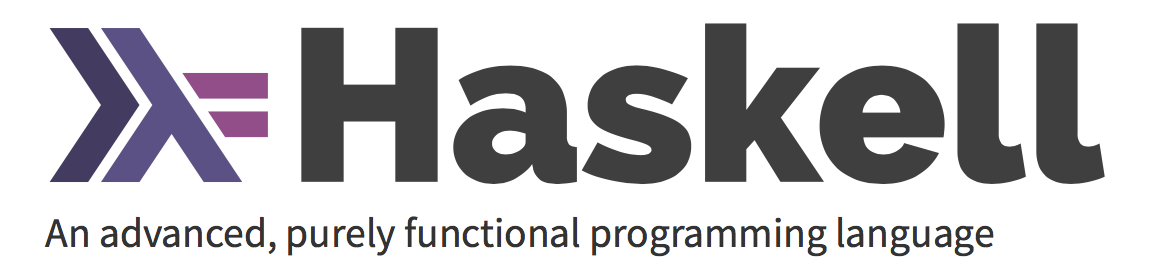/ FUNCTIONAL### 编程范式：

• 命令式编程 (Imperative programming)
• 声明式编程(Declarative programming)
1. 命令式编程 （Imperative programming）
将程序写成一条条指令，计算机依次执行得到最终结果，这种编写程序的方式叫做 命令式编程。 通常包含三种基本语句：
• 运算语句
• 循环语句
• 流程控制语句

1. 声明式编程（Declarative programming）

#### 声明式与命令式编程理念和风格

• 命令式编程是行动导向（Action-Oriented）的，因而算法是显性而目标是隐性的；
• 声明式编程是目标驱动（Goal-Driven）的，因而目标是显性而算法是隐性的。

Haskell 与其他语言不同，是一门纯粹函数式编程语言 (purely functional programming language)

2. Haskell 是静态类型 (statically typed) 的。当你编译程序时，编译器需要明确哪个是数字，哪个是字符串。

``````函数带有副作用指的是，函数的行为受系统的全局状态所影响。

``````

3. 新建脚本文件，以’.hs’结尾， 打开控制台输入 ghci，进入交互模式。
4. 运行 ‘:l xxx.hs’ 加载脚本，如果更新文件需 运行 ‘:r xxx.hs’

List 是一种单类型的数据结构，可以用来存储多个类型相同的元素。List 中的 List 可以是不同长度，但必须得是相同的类型.

`head` 返回一个 List 的头部，也就是 List 的首个元素。
`tail` 返回一个 List 的尾部，也就是 List 除去头部之后的部分。
`last` 返回一个 List 的最后一个元素。
`init` 返回一个 List 除去最后一个元素的部分。
`reverse` 将一个 List 反转:
`take` 返回一个 List 的前几个元素

#### List Comprehension 列表推导式

``````ghci> [x*2 | x <- [1..10]]
[2,4,6,8,10,12,14,16,18,20]
``````

#### Tuple 元组

1. Tuple 中的项由括号括起，并由逗号隔开。
2. 要求对需要组合的数据的数目非常的明确。
3. 它的类型取决于其中项的数目与其各自的类型。

1. 把所有三遍长度小于等于 10 的三角形都列出来。 从三个 List 中取值，并且通过输出函数将其组合为一个三元组
``````let triangles = [ (a,b,c) | c <- [1..10], b <- [1..10], a <- [1..10] ]
``````

1. 添加一个限制条件，令其必须为直角三角形。同时也考虑上 b 边要短于斜边，a 边要短于 b 边情况：
``````let rightTriangles = [ (a,b,c) | c <- [1..10], b <- [1..c], a <- [1..b], a^2 + b^2 == c^2]
``````
2. 修改函数，告诉它只要周长为 24 的三角形
``````let rightTriangles' = [ (a,b,c) | c <- [1..10], b <- [1..c], a <- [1..b], a^2 + b^2 == c^2, a+b+c == 24]
``````

调用 函数 `rightTriangles`

### 函数的合成与柯里化

`f(x)``g(x)`合成为`f(g(x))`，有一个隐藏的前提，就是`f``g`都只能接受一个参数。如果可以接受多个参数，比如`f(x, y)``g(a, b, c)`，函数合成就非常麻烦。 这时就需要函数柯里化了。所谓”柯里化”，就是把一个多参数的函数，转化为单参数函数。